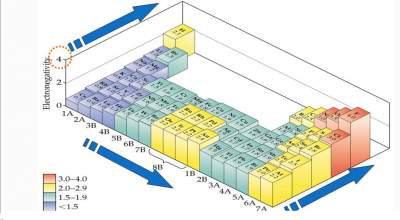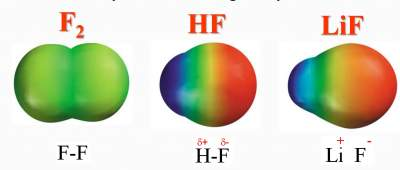# Bond Polarity and Electronegativity

## Introduction

A Covalent Bond may be:
• Polar: when electrons in the bond are attracted to one of the atoms. Example: H-F bond
• Non-polar: when electrons are equally shared between atoms along the bond. Example: F-F bond
Polarity of the bond depends on the ability of one atom to attract the electrons of the bonds towards it more than the other atom → Electronegativity.

### Electronegativity:

• he ability of an atom in a molecule to attract electrons to itself.
• The difference in electronegativity between the two elements sharing a covalent bond indicates its polarity .
• Hence, the trends of the "Electronegativity" in the Periodic TableThe "Electronegativity" scale.

### Trends of the "Electronegativity" in the Periodic Table• The highest electronegative element is F (4.0)
• The lowest electronegative element is Cs (0.7)

### Examples

#### F2molecule (F-F)

• Difference is 4.0 − 4.0 = zero
• → Electrons are equally shared between the 2 F atoms
• Non-polar covalent bond

#### HF molecule (H-F)

• Difference is 4.0 − 2.1 = 1.9
• → Electrons are more attracted towards F (higher electronegative)
• Polar covalent bond

#### LiF molecule (Li-F)

• Difference is 4.0 − 1.0 = 3.0
• → Electrons are very attracted towards F
• Ionic bondPolarity of the bond is measured by the Dipole Moment (μ) = Q r
Where:
• μ = dipole moments
• Q = charge (in coulombs (c)
• r = distance between 2 charges (in m)
• The unit of μ called debye (D) where 1 D = 3.34 × 10− 30 coulomb-meter

### Exercises on Bond Polarity and Electronegativity

Exercise I on Calculating the Dipole Moment (μ)

Check your answers here: Solution to the Exercise I on Calculating the Dipole Moment (μ

Exercise II on Calculating the Dipole Moment (μ)

Check your answers here: Solution to the Exercise II on Calculating the Dipole Moment (μ

Exercise III on Calculating the Dipole Moment (μ)

Check your answers here: Solution to the Exercise III on Calculating the Dipole Moment (μ)

### Relation between Bond Polarity & Bond Distance

Explanations:
• Bond electrons tend to be more on the bond as the difference in electronegativity between H and each of the halogens decreases.
• Size of the halogens increases as their atomic numberincreases; from top (F) to bottom (I)

For more details, please contact me here.
Date of last modification: Summer , 2019